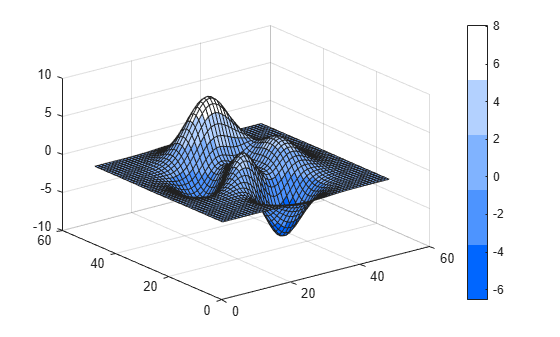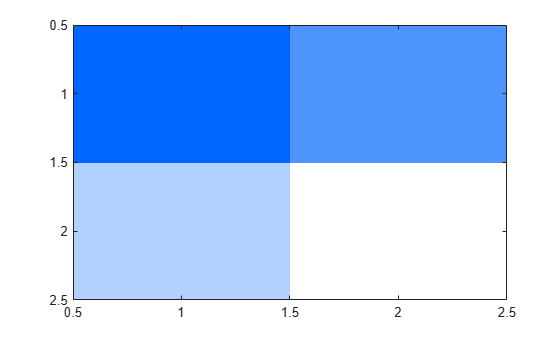Main Content

# hsv2rgb

Convert HSV colors to RGB

## Syntax

``RGB = hsv2rgb(HSV)``
``rgbmap = hsv2rgb(hsvmap)``

## Description

example

````RGB = hsv2rgb(HSV)` converts the hue, saturation, and value (HSV) values of an HSV image to red, green, and blue values of an RGB image.```

example

````rgbmap = hsv2rgb(hsvmap)` converts an HSV colormap to an RGB colormap.```

## Examples

collapse all

Create a three-column HSV matrix that specifies five shades of blue. In this case, hue and value are constant, while saturation varies between `1.0` and `0.0`.

`hsv = [.6 1 1; .6 .7 1; .6 .5 1; .6 .3 1; .6 0 1]; `

Convert the HSV matrix to a colormap by calling `hsv2rgb`. Then use that colormap in a surface plot.

```rgb = hsv2rgb(hsv); surf(peaks); colormap(rgb); colorbar```Create a 2-by2-by-3 HSV array that specifies four shades of blue.

```hsv(:,:,1) = ones(2,2)*.6; hsv(:,:,2) = [1 .7; .3 0]; hsv(:,:,3) = ones(2,2);```

Convert the HSV array to a truecolor image using `hsv2rgb`. Then display the image.

```rgb = hsv2rgb(hsv); image(rgb);```## Input Arguments

collapse all

HSV image, specified as an m-by-n-by-3 numeric array with values in the range [0, 1]. The third dimension of `HSV` defines the hue, saturation, and value for each pixel, respectively, as described in the table.

AttributeDescription
HueValue from `0` to `1` that corresponds to the color’s position on a color wheel. As hue increases from `0` to `1`, the color transitions from red to orange, yellow, green, cyan, blue, magenta, and finally back to red.
SaturationAmount of hue or departure from neutral. `0` indicates a neutral shade, whereas `1` indicates maximum saturation.
Value

Maximum value among the red, green, and blue components of a specific color.

Data Types: `double` | `single` | `logical`

HSV colormap, specified as a c-by-3 numeric matrix with values in the range [0, 1]. Each row of `hsvmap` is a three-element HSV triplet that specifies the hue, saturation, and value components of a single color of the colormap.

Data Types: `double`

## Output Arguments

collapse all

RGB image, returned as an m-by-n-by-3 numeric array with values in the range [0, 1]. The third dimension of `RGB` defines the red, green, and blue intensity of each pixel, respectively. The image has the same data type as the HSV image, `HSV`.

Data Types: `double` | `single`

RGB colormap, returned as a c-by-3 numeric matrix with values in the range [0, 1]. Each row of `rgbmap` is a three-element RGB triplet that specifies the ref, green, and blue components of a single color of the colormap. The colormap has the same data type as the HSV colormap, `hsvmap`.

Data Types: `double` | `single`

 Smith, A. R. “Color Gamut Transform Pairs”. SIGGRAPH 78 Conference Proceedings. 1978, pp. 12–19.

Download ebook